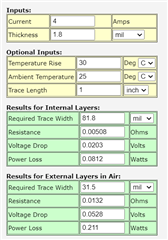If you have a related question, please click the "Ask a related question" button in the top right corner. The newly created question will be automatically linked to this question.

# SN74LVC1G123: How does RL included into calculation of pulse duration ?

Part Number: SN74LVC1G123
Other Parts Discussed in Thread: CD40109B, UCC27517

Hi,

The pulse duration I need to generate is 0.1us to 10us.

If 1000pF is used as  Cext  , and the RL is 1kohm to 10kohm from figure 12 in datasheet. K is 1.11 if 3.3V supply is used (from figure 4.2)

Question:

• What is Rext ­used in this case ? tw = K x Rext X Cext  = 1.11 x Rext x 1000pF

Is it directly calculate Rext = tW / (K x Cext ) ?

• How RL calculation involved in this case ? Is it both Rext  and RL need to involved in this calculation?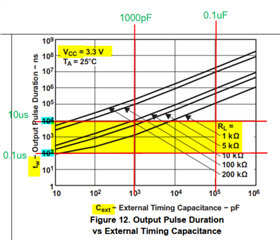• What is Rext ­used in this case ? tw = K x Rext X Cext  = 1.11 x Rext x 1000pF

Is it directly calculate Rext = tW / (K x Cext ) ?

Yes, that is correct.

How RL calculation involved in this case ? Is it both Rext  and RL need to involved in this calculation?

RL is not part of the calculation for the pulse width. RL is the load resistance at the output, and is representative of the circuit connected at the output of the device. We use it for testing -- generally speaking you wouldn't add a resistor at the output of a standard logic device.

• Thanks you Maier.

• Hi Maier,

May i know what is the rise and fall time of the generated output ?

Does it affected by the Rext and Cexr also ?

If the amplitude i generated is higher (12V), does it cause the rise/fall time of small pulse duration (0.1us) does not have enough time to rise to high amplitude (12V) ?

• The SN74LVC1G123 is only designed to operate between 1.65V and 5.5V - it will not work at 12V.

The output transition time will typically be just a few nanoseconds into a standard 50 pF load.

• Ya. Noted on the output until 5.5V. I use NPN to level shifter up to 12V.

Can i say that the output transition is depend on the probe be used ?

• Yes - we have a detailed explanation on calculating output transition rates located here:

(+) [FAQ] What is the output transition rate for a logic device? - Logic forum - Logic - TI E2E support forums

• ok. thank you for your information.

• Hi Maier,

Based on the link, if the supply is 3.3V, and I want to get 2.7V-3V output VOH , I need to use lower resistance for ROH ?

or i cannot use lower resistor than 30ohm ? due to the output high current 24mA.

If I use 1kohm for RL , can I get max 0.55V for VOL and min 2.3V for VOH ?

• ROH is the internal resistance of the device - it is not a resistor you add.

Don't add any resistance to the output - just connect the device to the input of your 12V conversion circuit.

• May i know the output will be min 2.3V for VOH at 3.3V supply ?

With the circuit you showed me, I expect the output current to be 10.1mA: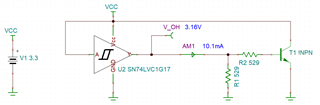Using that value, the worst case VOH would be found by:

(1) determine the output resistance at 3.3V -- the closest in the datasheet is 3V, so we'll use that.

ROH = (VCC - VOH) / IOH = (3 - 2.4) / 0.016 = 37.5 ohms

(2) use the calculated resistance to determine the worst-case voltage drop from VCC at the given current:

V_drop = IOH * ROH = 37.5*0.0101 = 0.37875 V

(3) subtract the drop from the supply voltage

VOH = VCC - V_drop ~= 2.92V

So, your worst-case expected output high-state voltage is 2.92V, and the typical output high-state voltage will be about 3.16V.

• i have changed the circuit to NMOSFET. The pull low change to 1kohm.

The IOH above is 0.016. how does this value calculated ?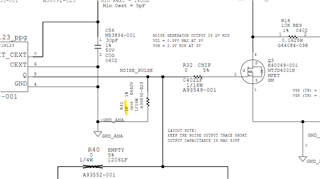• I showed every step above - please follow what I did yourself and do the calculations.

• Because I saw there is 2 condition for IOH = 16mA for VOH = 2.4V ; and IOH = 24mA for VOH = 2.3V.

I not sure which is the IOH I should refer.

From the calculation shown, the higher the pull down I put, the VOH is higher and near the VCC.

Please correct if I am wrong.

• Current through a resistor is determined by Ohm's Law: I = V/R

If you put a 1kohm pull-down resistor at the output of the device, the maximum current at the output will be:

I = 3.3 / 1000 = 0.0033 A = 3.3 mA

A larger pull-down resistor will result in less current, for example, changing to a 100kohm resistor:

I = 3.3 / 100000 = 0.000033 A = 33 uA

Lower current will result in less voltage drop (V_drop) and a larger V_OH.

• Ok. understand .

When to determine IOH = 16mA for VOH = 2.4V ; and IOH = 24mA for VOH = 2.3V ? as shown in datasheet

• If you have a 3V supply and 16mA output current, then use VOH(min) = 2.4V. If you have a 3V supply and 24mA output current, then use VOH (min) = 2.3V.

Generally speaking, I use the lower value for low currents ( < 16 mA ) and the large value when I need higher currents ( > 16mA )

• so the output current 24mA and 16mA is the guideline for the min VOH ?

the actual VOH is determined by the output resistance. Am I correct ?

• That is correct - the VOH value will be directly related to the output current and the resistance of the high-side MOSFET. The output structure is shown below, and you can see how there is a voltage drop between VCC and Vout: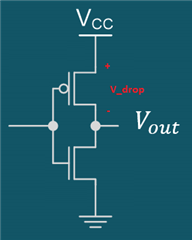V_drop is the value I gave in my post above, which is just IOH * ROH, with ROH being the resistance of the FET.

• Thanks Maier for your explanation. I understand that.

• I'm happy to help. Please let me know if you need additional assistance.

• Hi Maier,

If pull down resistance change to 10kohm, and the supply is 3.3V.

IOH = 3.3/ 10k = 0.33mA

Use 2.4V as IOH < 16mA

ROH = (3-2.4) / 0.016 = 37.5 ohm

Vdrop = 0.33 x 37.5 = 12.375 V

VOH = VCC - Vdrop  = 3.3 - 12.375 = - 9.075 V

IOL = 0.55/10k = 55uA

ROL = 0.55 / 0.016 = 34.37 ohm

VOL = 55uA x 34.37 = 1.89mV

Is the calculation correct as VOH is -ve value ?

• Hi Maier,

Wrong calculation above.

If pull down resistance change to 10kohm, and the supply is 3.3V.

IOH = 3.3/ 10k = 0.33mA

Use 2.4V as IOH < 16mA

ROH = (3-2.4) / 0.016 = 37.5 ohm

Vdrop = 0.33m x 37.5 = 0.01237V

VOH = VCC - Vdrop  = 3.3 - 0.01237 = 3.287V

IOL = 0.55/10k = 55uA

ROL = 0.55 / 0.016 = 34.37 ohm

VOL = 55uA x 34.37 = 1.89mV

Does it correct ?

• Yes, that is correct.

• thanks for confirmation. Maier.

• Does CD40109B able to level shift it up to 12V ? I saw there is long propagation delay time. Does it suitable to level shift up the output from SN74LVC1G123 to 12V while maintaining the pulse duration [0.1us (min) to 10us (max)] ?

• Yes - CD40109B can support 3V to 18V up-translation, which also includes 3.3V to 12V up-translation.

The delays are fairly long - with a 3.3V input supply, I would expect greater than 600 ns of delay, which could cause severe distortion or even missing the 0.1us pulse entirely.

The easiest way to achieve this is with an nFET inverter, which I believe was your plan. The pull-up resistor value and parasitic capacitance on the output will be the primary limiting factors on how fast the inverter will operate.

To give you an equation to work with - the rise time of an RC circuit (10% to 90%) is approximately 2.2 * R * C -- so to get a fast rising edge (let's say 100ns) given a typical 50pF load:

R = 100n / (2.2*50p) = 909 ohms

So, a 1kohm resistor should suffice to have a pretty fast edge -- you could go with 330 ohms to be even faster, depending on your system requirements.

• This is my circuitry for the NMOS inverter. I use 100kohm for 1st NMOS pull up and 200kohm for 2nd NMOS pull up. This is because the low side of output is high (>9V) if I use 1kohm or 10kohm pull up. With these combination, I get ~1V VOL and ~11V for VOH for the output of 2nd NMOS.

The value of pull up I used is contradict with your equation of rise time for RC circuit.

The switching of NMOS I used is td(on) = 2.5ns, tr = 1ns, td(off) = 16ns, tr = 8ns.

Will it affected by the equation you mentioned ?

By the way, is there any other voltage translator up to 12V and have much lower propagation delay ?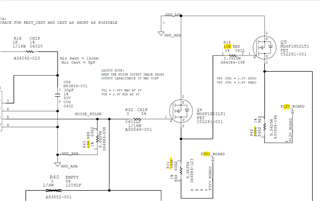• We don't have any other 12V capable translators in TI, but there are other devices that can support 12V operation. For example, opto-isolators can be used to convert from 3.3V to 12V, but for this kind of circuit I would consider it overkill.

From the datasheet of your nMOS: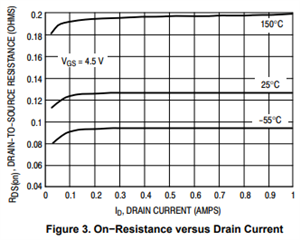The on-state resistance is extremely low, so you shouldn't be seeing any issues with using a 1k pull-up resistor on both stages.

• if I used this 100k and 200k pull up resistor, it might cause the slow rising edge of the output ?

• Yes, from the equation I provided previously, you can expect the output edges with a 50pF load to rise in approximately:

2.2 * 100k * 50p = 11 us

Which is much too slow for your desired 0.1us pulse width.

• Hi Maier,

Thanks for your hints. By changing the pull up for 3.3V rail and 12V rail, I manage to get ~0.1us pulse output from NOMOS.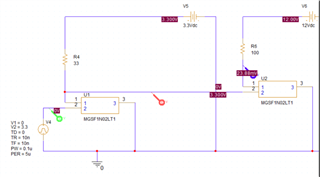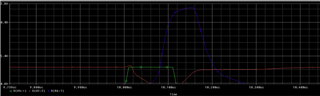Do you have the simulation model for SN74LTC1G123  that shown in your snapshot above? Could you share ?• is there any concern if only using 0.25W rating for both pull up resistor ? especially for 12V voltage rail.

• Power is calculated by P = IV, or in this case, P = V^2/R

Resistors should have at least the rating to handle the power used.

• I use 100ohm pull up at 0.25W rating. The power used is 1.44W

I changed to 330ohm at 0.5W rating. But the max amplitude is slightly lower than 12V.

• If you want to get 100 ohm equivalent, then I would recommend to put 10 1k resistors in parallel. This will result in a 100 ohm equivalent resistance, but the power is spread out across 10 devices instead of only 1, which means each only has to provide 144 mW. This would allow using standard 0.25 W resistors.

• However, the resistor size is too big for my board if use 10 pcs of 1k resistor in 1206 package.

By the way, do yo have the simulation model for SN74LTC1G123  that shown in your snapshot above? Could you share ?• To convert a 3.3 V signal to 12 V without pull-up/-down resistors, you can also use a gate driver with TTL-compatible input, such as the UCC27517.

• To convert a 3.3 V signal to 12 V without pull-up/-down resistors, you can also use a gate driver with TTL-compatible input, such as the UCC27517.

Thanks Clemens, that's a good idea.

I tend to get caught up only thinking about the parts I support.

• hi Maier,

If use UCC27517 to level shift up the output from SN74LVC1G123, R1 and Q1 are needed to be used to switch the output ?

Or i can directly output the pulse without R1 and Q1 ?• The UCC27517 is designed as a gate driver; Q1 is an example of a device with a gate. The purpose of R1 is to reduce the drive strength to prevent Q1 from switching too fast.

You do not need Q1. You might need R1 if the extremely fast output edges generate EMI problems.

• May I know how much R1? If the output trace is left floating in order to couple 200mV noise to PCIe lane. The trace length is ~ 11 inch.

• If you are using the output trace as an antenna, then its characteristics depend on the board layout. You will have to experiment. (But if the purpose is to generate EMI, 0 Ω might work.)

• By using Pspice simulation, 10kohm series resistor is used and there is ~0.8V overshoot and undershoot with 12V amplitude output. Any recommendation value to reduce this overshoot / undershoot ?

• Is overshoot actually a problem in this application?

Anyway, I doubt that it is possible to use Pspice to accurately model the characteristics of the board. You have to to actual experiments.

• ok. understand. Thanks.

• if R1 is needed, then high power rating resistor is needed ? due to high power dissipation.

• The power dissipation in the resistor is, in theory, (12 V)² / R1, but with a trace capacitance of about 30 pF, only for a very short time. I do not think you need to care about that.

• what is the recommended trace width for the output ?

• if make the trace width to 31.5mil, then the

PCB trace Capacitance / length   = ε0 εrw / h

= 0.225pF/inch x 3.4 x 31.5 mil / 2.85 mil

= 8.455 pF/inch

The total trace length as the antenna is ~11inch. Then the PCB trace capacitance = ~93pF.

With this capacitance, it will cause the rise/fall time of the pulse width to increase, which is not preferred in my case here.

What is the recommendation in this case in order to have 12V amplitude with ~12ns rise/fall time for 0.1us pulse duration output ?

Can I have only <20mils trace length for 31.5mil trace width and 3.5mil trace width for the remaining trace length ?